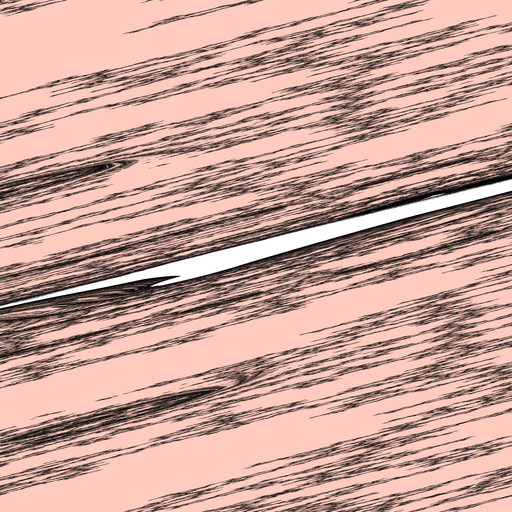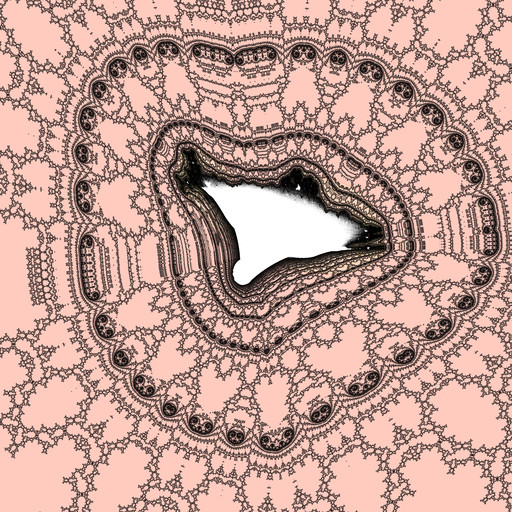# mathr

## Unskewing the Burning Ship

The Burning Ship fractal is interesting because it has mini-ships among filaments. However, some of these filaments and mini-ships are highly stretched or skewed non-conformally, which makes them look ugly. Some fractal software (Kalles Fraktaler, Ultrafractal, ...) has the ability to apply a non-uniform scaling to the view, which unstretches the filaments and makes (for example) the period-doubling features on the way to the mini-ship appear more circular. However, to my knowledge, all these software require manual adjustment of the unskewing transformation, for example dragging control points in a graphical user interface.

The size estimate for the Burning Ship mini-sets is based on the size estimate for the Mandelbrot set. The Burning Ship iterations are not a well behaved complex function, so Jacobian matrices over real variables are necessary. Generic pseudo-code for the size estimate of an arbitrary function:

d := degree of smallest non-linear power in f
a, b := coordinates of a mini-set with period p
x, y := critical point of f (usually 0, 0)
L := I
B := I
for j := 1 to p - 1
x, y := f(x, y)
L := Jf(x, y)
B := B + L^{-1}
size := 1 / sqrt(abs(det(L^(d/(d-1)) B)))

In particular for the power 2 Burning Ship this resolves to:

d := 2
x := 0
y := 0
L(lxx, lxy; lyx, lyy) := (1, 0; 0, 1)
B(bxx, bxy; byx, byy) := (1, 0; 0, 1)
for j := 1 to p - 1
x, y := (x^2 - y^2 + a, abs(2 x y) + b)
L := ( 2 x lxx - 2 y lyx
, 2 x lxy - 2 y lyy
, 2 sgn(x) sgn(y) (x lyx + lxx y)
, 2 sgn(x) sgn(y) (x lyy + lxy y)
)
detL := lxx * lyy - lxy * lyx
B := ( bxx + lyy / detL
, bxy - lyx / detL
, byx - lxy / detL
, byy + lxx / detL
)
detL := lxx * lyy - lxy * lyx
detB := bxx * byy - bxy * byx
size := 1 / sqrt(abs(detL^2 * detB))

Note the renormalization of the $$c=a+bi$$ plane by:

$L^\frac{d}{d - 1} B$

The size estimate takes out the uniform scaling factor from this 2x2 matrix, but it's also possible to use it to extract rotation and non-uniform scaling (stretching, skew) parameters. A problem comes when trying to raise the matrix $$L$$ to a non-integer power when $$d > 2$$, so I fudged it and hoped that the non-conformal contribution from $$L$$ is non-existent, and just used $$B$$. To cancel out the uniform scaling from $$B$$, I divide by the square root of its determinant, and I take its inverse to use as a coordinate transformation for the $$c=a+bi$$ plane:

$T = \left(\frac{B}{\sqrt{\det B}}\right)^{-1}$

I tested it briefly and it seems to work for Burning Ship power 2 and 3, and also Mandelbar/Tricorn power 2. There is a problem however: to get this automatic skew matrix, one needs to zoom deep enough so that Newton's method can find a mini-set, but doing that is tricky in hard-skewed areas. So I still need to write GUI code for manual skew, to make that pre-navigation easier.

Here's just one image pair, power 2 Burning Ship, the first is with an identity transformation, the second with automatically calculated unskewing transformation:One last thing: it should be possible to separate skew from rotation by applying polar decomposition.